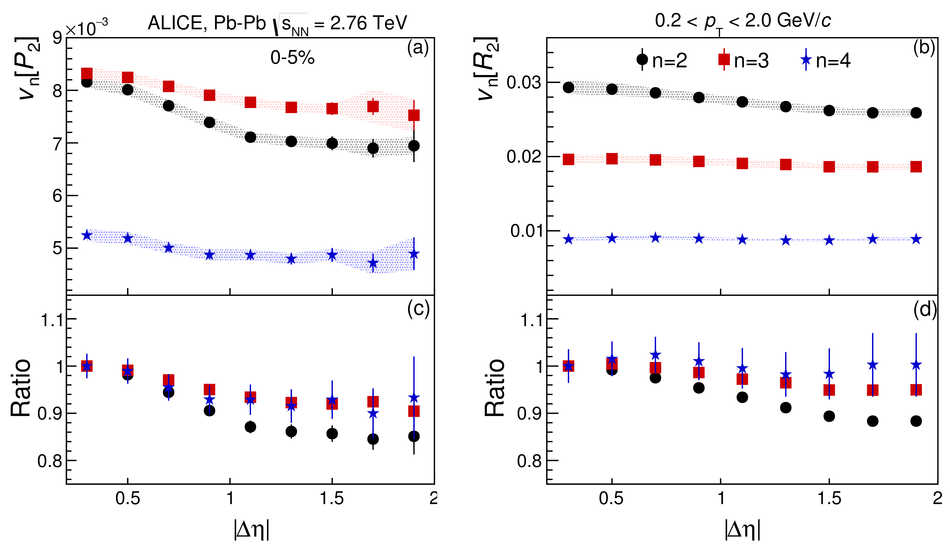Flow dominance and factorization of transverse momentum correlations in Pb-Pb collisions at the LHC

We present the first measurement of the two-particle transverse momentum differential correlation function, $P_2\equiv\langle \Delta p_{\rm T} \Delta p_{\rm T} \rangle /\langle p_{\rm T} \rangle^2$, in Pb-Pb collisions at $\sqrt{s_{_{\rm NN}}} =$ 2.76 TeV. Results for $P_2$ are reported as a function of relative pseudorapidity ($\Delta \eta$) and azimuthal angle ($\Delta \varphi$) between two particles for different collision centralities. The $\Delta \phi$ dependence is found to be largely independent of $\Delta \eta$ for $|\Delta \eta| \geq$ 0.9. In 5% most central Pb-Pb collisions, the two-particle transverse momentum correlation function exhibits a clear double-hump structure around $\Delta \varphi = \pi$ (i.e., on the away side), which is not observed in number correlations in the same centrality range, and thus provides an indication of the dominance of triangular flow in this collision centrality. Fourier decompositions of $P_2$, studied as a function of collision centrality, show that correlations at $|\Delta \eta| \geq$ 0.9 can be well reproduced by a flow ansatz based on the notion that measured momentum correlations are strictly determined by the collective motion of the system.

Figures

Figure 1

 (a) $P_2 (\Deta,\Dphi)$ in the 5% most central Pb-Pb collisions. The region $|\Deta| < 0.15$ and $|\Delta\varphi| < 0.13$ rad, where the weight technique used in this analysis does not provide a reliable efficiency correction, is excluded. (b) $P_2 (\Dphi)$ for $|\Delta\eta| \geq 0.9$ Systematic errors are shown as gray boxes. Note the statistically significant dip at $\Delta\varphi \sim \pi$.Figure 2

 $v_{n}$ coefficients where $n=2,3,4$ in the range (a) $0.2 \leq |\Delta\eta| \leq 0.9$ and (c) $0.9 \leq |\Delta\eta| \leq 1.9$ obtained from the $P_2$ correlation function. The coefficients are compared with the expectations from the flow ansatz calculated in their respective $\Deta$ ranges in Pb-Pb collisions. Statistical errors are shown as vertical solid lines, whereas systematic errors are displayed as colored bands Ratios of the $v_{n}$ coefficients and their corresponding flow ansatz values are shown in (b) and (d). The errors on the ratios are only statistical.Figure 3

 $v_{n}$ coefficients, $n=2, 3, 4$, obtained from (a) $P_2$ and (b) $R_2$ correlators, as a function of $|\Delta\eta|$ in the 5% most central Pb - Pb collisions Statistical errors are shown as vertical solid lines whereas systematic uncertainties are displayed as shaded bands. Panels (c - d): ratios of the $v_{n}$, $n=2, 3, 4$, by the corresponding values of $v_{n}$ measured at $\Delta\eta = 0.3$.